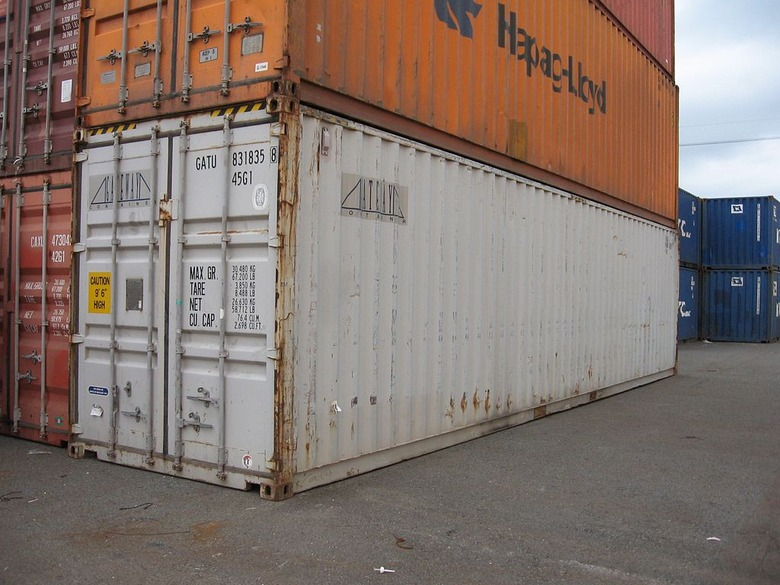# Identifying Nets For Cubes# Introduction to Nets

Watch the video. Then, discuss the following concepts.

• The 2-D version of a cube is called a net.
• The surface area of a prism is the area of its net.
• You can find the surface area of a 3-D figure by adding together the areas of each surface.
• The surface area of a cube with side lengths of 1 unit is 6 square units.

VIDEO: Cube

1 of 9